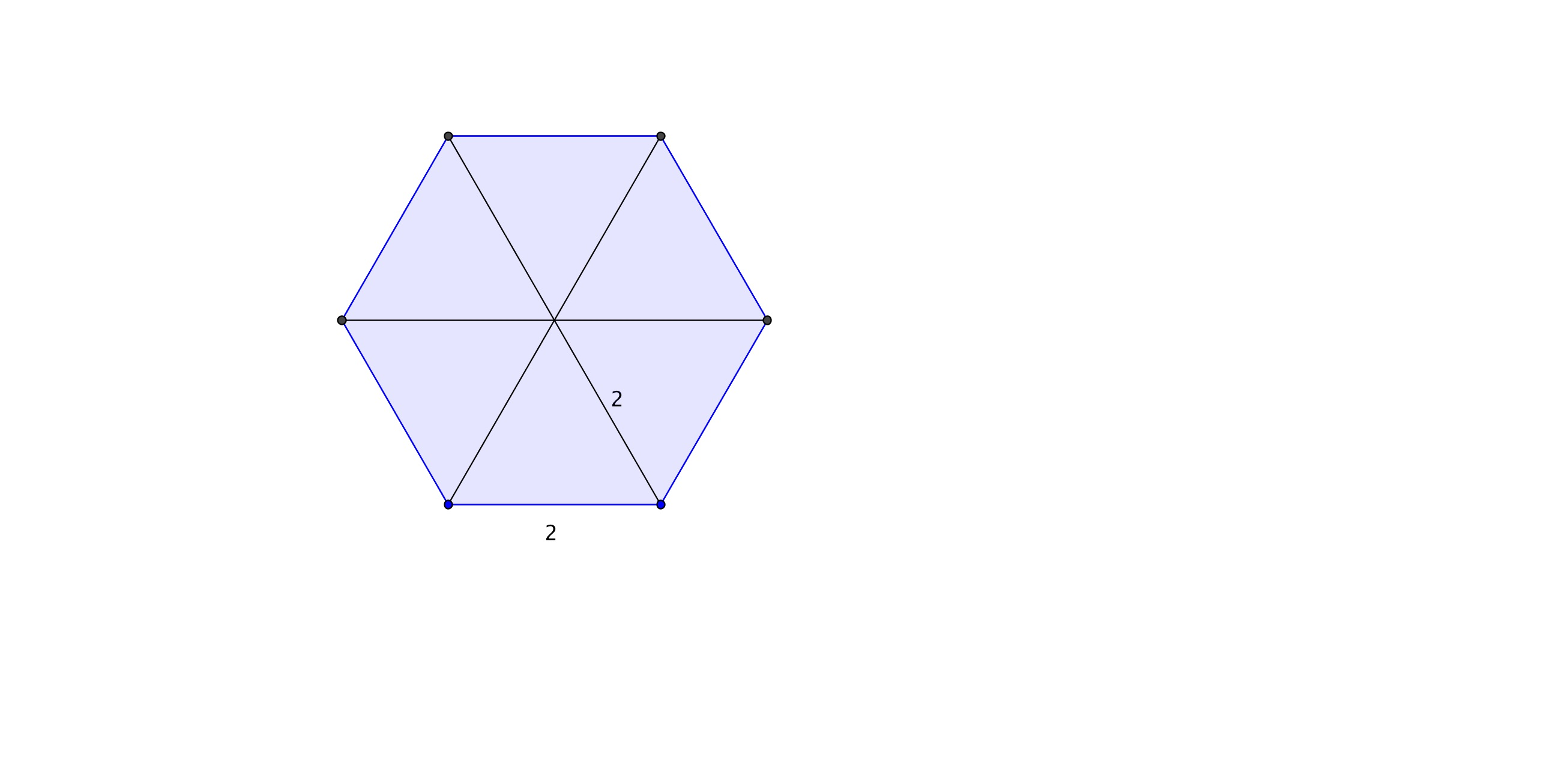# GEOM 1 | Lesson 2 | Explore (Area of Polygons) Solutions

Use your knowledge of perimeter and area developed in Lesson 1 to answer the following.

1. A segment has been drawn on the hexagon. Use the given line segment to construct a set of equilateral triangles in the given hexagon.1. Find the perimeter of this hexagon using the properties of equilateral triangles.  12

1. A regular polygon is defined to be a polygon where all angles and sides are congruent. Given this definition is the hexagon above regular?  How do you know?  yes

1. Using your knowledge of area of triangles, calculate the area of the hexagon?  Area of each triangle is $$\dfrac{1}{2}bh$$